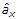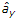# Electronics and Communication Engineering - Electromagnetic Field Theory

6.
Radiation resistance of half-wave dipole (λ/2) antenna is in free space
73 Ω
120 p
36.5 Ω
70 Ω
Explanation:
No answer description is available. Let's discuss.

7.
The effect of the inductance of a coil on a constant direct current is that
it does not effect the constant direct current
it decreases the current
it strengthens the current
it causes a higher voltage drop
Explanation:
No answer description is available. Let's discuss.

8.
If H = 0.1 sin (108p t + βλ)A/m for a plane wave propagating in free space, then the time average Poynting vector is
(0.6psin2 βy)w/m2
- 0.6pw/m2
1.2pw/m2
- 1.2pw/m2
Explanation:
No answer description is available. Let's discuss.

9.
The ratio of tangential component of electric field at the surface of a conductor and the linear current density resulting from this field is __________ impedance
characteristic
critical
surface
polarizing